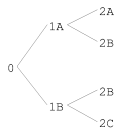## 18 Graph Formatting

• 18.1 Graph Formatting Functions
• 18.2 The Graph Formatting Protocols
• CLIM provides a mechanism for arranging arbitrary output in a graph. The following code produces the graph shown in Figure 18.1. [annotate]

```(defun graph-test (stream &optional (orientation :horizontal))
(fresh-line stream)
(macrolet ((make-node (&key name children)
`(list* ,name ,children)))
(flet ((node-name (node)
(car node))
(node-children (node)
(cdr node)))
(let* ((2a (make-node :name "2A"))
(2b (make-node :name "2B"))
(2c (make-node :name "2C"))
(1a (make-node :name "1A" :children (list 2a 2b)))
(1b (make-node :name "1B" :children (list 2b 2c)))
(root (make-node :name "0" :children (list 1a 1b))))
(format-graph-from-roots
(list root)
#'(lambda (node s)
(write-string (node-name node) s))
#'node-children
:orientation orientation
:stream stream)))))
```Figure 18.1: Example of graph formatting.